Home > INT3 > Chapter 7 > Lesson 7.2.5 > Problem7-116

7-116.

Solve each equation. Homework Help ✎

1. log5(2x) = 3

2. log5(x + 1) = –1

3. log(4) – log(x) = 2

4. 2log3(6) + log3(y) = 4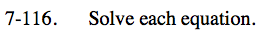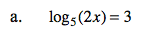53 = 2x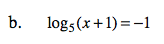5−1 = x + 1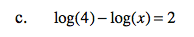$\log_{10} \left (\frac{4}{x} \right )=2$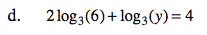log3(62) + log3(y) = 4